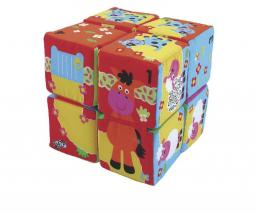# Area of a cube

Calculate the surface area of a cube if its volume is equal to 729 cubic meters.

S =  486 m2

### Step-by-step explanation:Did you find an error or inaccuracy? Feel free to write us. Thank you!Tips to related online calculators
Tip: Our volume units converter will help you with the conversion of volume units.

## Related math problems and questions:

• The volume 2The volume of a cube is 27 cubic meters. Find the height of the cube.
• Cube V2SThe volume of the cube is 27 dm cubic. Calculate the surface of the cube.
• Cube surface and volumeFind the surface of the cube with a volume of 27 dm3.
• Cube surface and volumeThe surface of the cube is 500 cm2, how much cm3 will be its volume?
• Cube surfce2volumeCalculate the volume of the cube if its surface is 150 cm2.
• The cubeThe cube has a surface area of 216 dm2. Calculate: a) the content of one wall, b) edge length, c) cube volume.
• Area to volumeIf the surface area of a cube is 486, find its volume.
• The cubeThe cube has a surface area of 486 m ^ 2. Calculate its volume.
• Cube wallThe perimeter of one cube wall is 120 meters. Calculate the surface area and the body diagonal of this cube.
• Volume and areaWhat is the volume of a cube which has an area of 361 cm2?
• Cube 6Volume of the cube is 216 cm3, calculate its surface area.
• The cubeThe cube has a surface of 600 cm2. What is its volume?
• Equilateral cylinderEquilateral cylinder (height = base diameter; h = 2r) has a volume of V = 199 cm3 . Calculate the surface area of the cylinder.
• Cube volumeThe cube has a surface of 384 cm2. Calculate its volume.
• TerezaThe cube has an area of base 256 mm2. Calculate the edge length, volume, and area of its surface.
• CylinderThe cylinder surface is 922 dm2. Its height is equal to the radius of the base. Calculate the height of this cylinder.
• Iron sphereIron sphere has weight 100 kg and density ρ = 7600 kg/m3. Calculate the volume, surface, and diameter of the sphere.